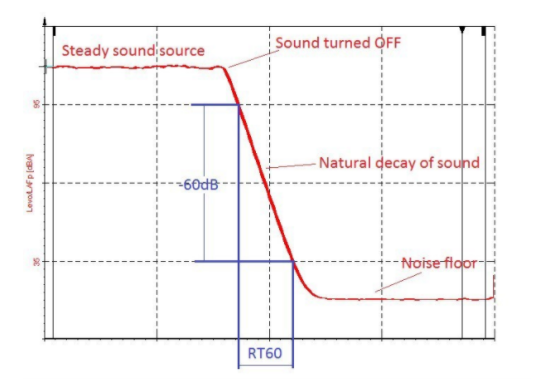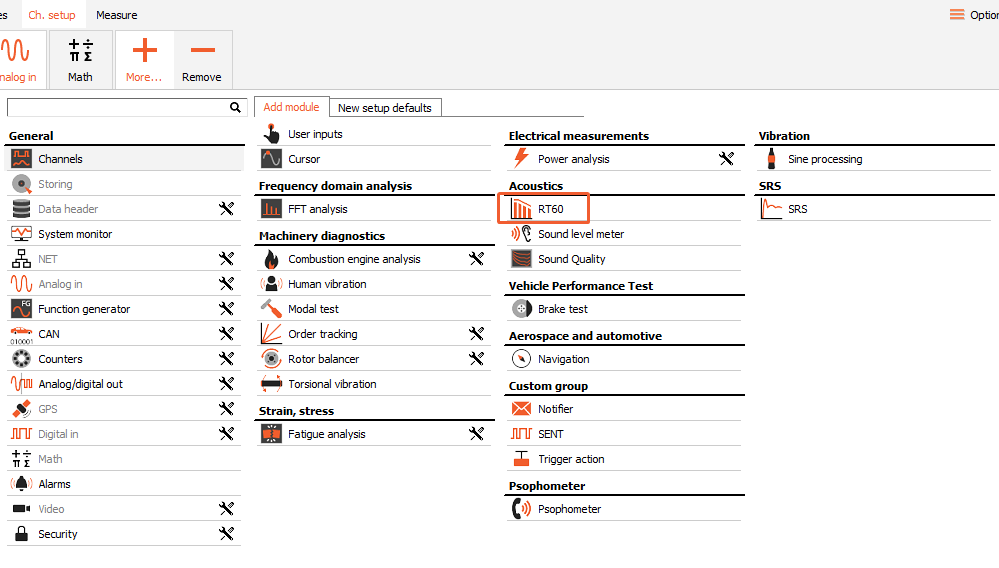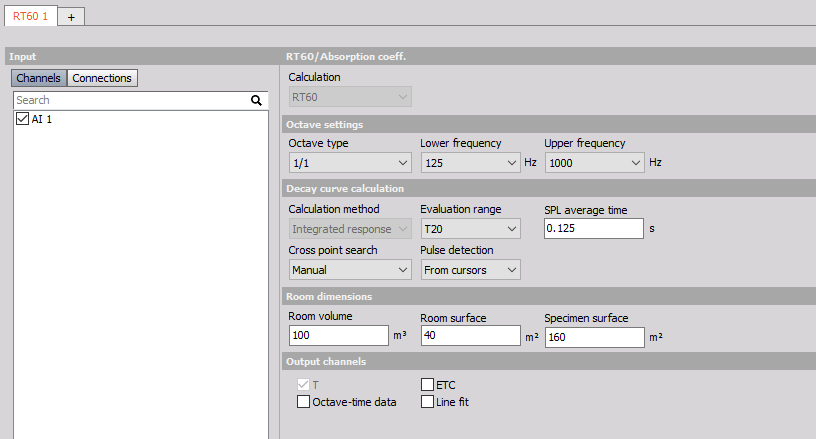Reverberation time is a time required for sound to decay 60 decibels from its initial level.Reverberation time is measured in narrow bands and differs depending on the frequency band being measured. For precision, it is important to know what ranges of frequencies are being described by a reverberation time measurement.

Rooms used for speech typically need a shorter reverberation time so that speech can be understood more clearly. If on the other hand, the reverberation time is too short, tonal balance and loudness may suffer . Reverberation effects are often used in studios to add depth to sounds. Reverberation changes the perceived spectral structure of a sound, but does not alter the pitch.

Basic factors that affect a room’s reverberation time include the size and shape of the enclosure as well as the materials used in the construction of the room. Every object placed within the enclosure can also affect the reverberation time.

## RT60 plugin setup in Dewesoft

RT60 plugin can be found in DEWESoft under Plugins section. Add a plugin with the click on it.The following window and setup appears when you add the plugin. First select the input channels (microphones)

The following window and setup appears when you add the plugin. First select the input channels (microphones).### Octave Settings

Octave analysis type can be selected from 1/1 or 1/3 octave. The difference is in the centre frequency and bandwidth of the bands. When defining the Lower frequency, we have to be careful, because of the longer wavelength of the sound with low frequency (the longest wavelength is conditioned with the room’s dimensions).

### Decay curve calculation

Decay curve is a graphical representation of the decay of the sound pressure level in a room as a function of time after the sound source has stopped. Integrated impulse response method is a method of obtaining decay curves by reverse-time integration of the squared impulse responses.

### Evaluation range

Reverberation time can be evaluated based on a smaller dynamic range than 60 dB and extrapolated to a decay time of 60 dB. The evaluation of the decay curve for each frequency band shall start at 5 dB below the initial sound pressure level. The bottom of the evaluation range should be at least 10 dB above the overall background noise of the measuring system.

• T10 is a time derived from the time at which the decay curve first reaches 5 dB and 15 dB below the initial level
• T15 is a time derived from the time at which the decay curve first reaches 5 dB and 20 dB below the initial level
• T20 is a time derived from the time at which the decay curve first reaches 5 dB and 25 dB below the initial level.
• T30 is a time derived from the time at which the decay curve first reaches 5 dB and 35 dB below the initial level.
• T60 is a time derived from the time at which the decay curve first reaches 5 dB and 65 dB below the initial level.

### SPL averaging type

Average time is a constant of an exponential averaging device and shall be less than 1/30 of the reverberation time.

### Cross point search

Option manual will calculate the reverberation time from the first to the second locked cursor.

Automatic search uses the improved algorithm for reverberation time estimation (Lundby algorithm). It is important to place the first cursor on the right position, and the position of the second cursor will be found automatically.

### Output Channels

• T – measured reverberation time
• ETC – energy time curve
• Octave-time data
• Line fit – makes a fitted curve on an energy time curve